Next: Scalar Einstein Equations Up: Evolution Equations Previous: Free Streaming

### Boltzmann Equations

The explicit form of the Boltzmann equations for the temperature and polarization follows directly from the Clebsch-Gordan relation of Eqn. (58). For the temperature (s=0),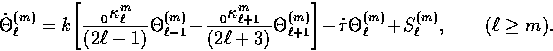(52)
The term in the square brackets is the free streaming effect that couples the-modes and tells us that in the absence of scattering power is transferred down the hierarchy when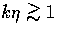. This transferral merely represents geometrical projection of fluctuations on the scale corresponding to k at distance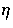which subtends an angle given by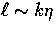. The main effect of scattering comes through the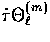term and implies an exponential suppression of anisotropies with optical depth in the absence of sources. The source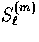accounts for the gravitational and residual scattering effects,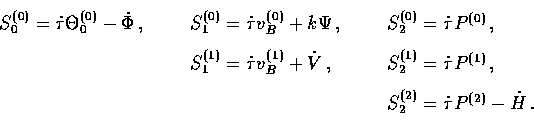(53)
The presence of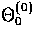represents the fact that an isotropic temperature fluctuation is not destroyed by scattering. The Doppler effect enters the dipole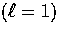equation through the baryon velocity vB(m) term. Finally the anisotropic nature of Compton scattering is expressed through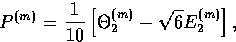(54)
and involves the quadrupole moments of the temperature and E-polarization distribution only.

The polarization evolution follows a similar pattern for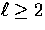,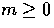from Eqn. (58) with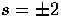,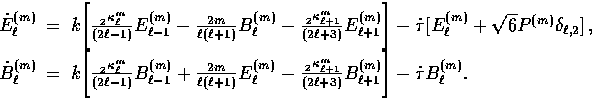(55)
Notice that the source of polarization P(m) enters only in the E-mode quadrupole due to the opposite parity of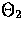and B2. However, as discussed in §IIB, free streaming or projection couples the two parities except for the m=0 scalars. Thus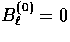by geometry regardless of the source. It is unnecessary to solve separately for the m=-|m| relations since they satisfy the same equations and solutions with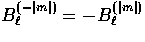and all other quantities equal.

To complete these equations, we need to express the evolution of the metric sources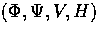. It is to this subject we now turn.Next: Scalar Einstein Equations Up: Evolution Equations Previous: Free Streaming
Wayne Hu
9/9/1997